TWiki >Rise Web>ClimbingRobot > ForceSensingAnkle>PreliminaryTests (30 Aug 2005, YongLaePark)

## Preliminary Tests for Multi Axial FSA Construction

Several preliminary tests were conducted to charaterize the deformation of the diaphragm. The main objectives of the tests are to identify the best places to put strain gages which maximize output signal and minimize undesired force detection (distinguishement between lateral forces and vertical forces).

### Test 1: Vertical Force Test on a Load Cell with Foil Strain Gages

Three foil strain gages are placed at three different spots on the diaphragm, and vertical forces are applied to the load cell as shown in Figure 1-1. Figure 1-2 shows the plot of output signals.

• Figure 1-1: Test Setup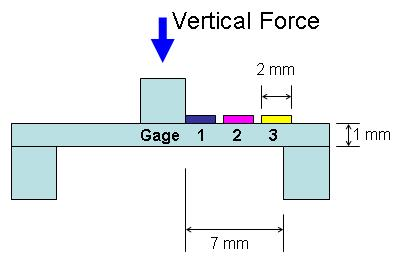• Figure 1-2: Voltage Output(mV) vs. Applied Force(N)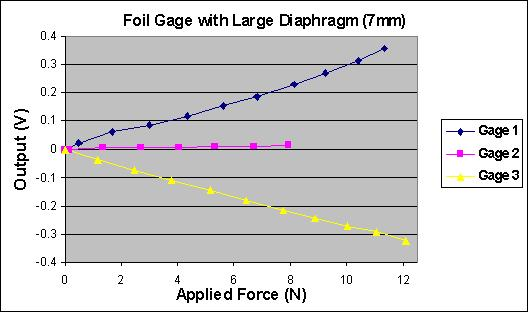Since the pink gage(gage 2) is placed almost on the center point of the diaphragm where little bending happens, it gives very small signal. However, gage 1 and 3 give big signal because bending happens somewhere close to both edges of the diaphragm. The resulting shape is shown in Figure 1-3.

• Figure 1-3: Resulting Shape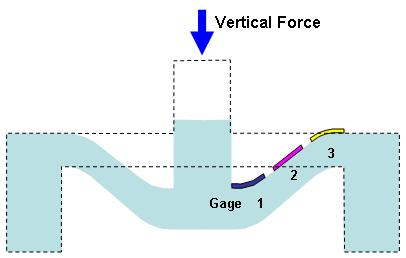The foil gages are large enough to cover the deforming area from the end of the diaphragm. Blue gage(gage 1) and yellow gage(gage 3) detect most deformation of the diaphragm.

### Test 2: Vertical Force Test on a Load Cell with Semiconductor Strain Gages

Three semiconductor strain gages are placed at three different spots on the diaphragm, and vertical forces are applied to the load cell as shown in Figure 2-1.

• Figure 2-1: Test Setup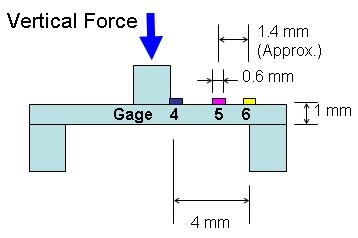• Figure 2-2: Voltage Output(mV) vs. Applied Force(N)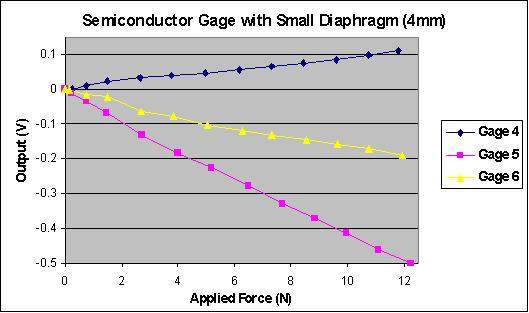Even though we checked most bending happens somewhere close to edges from the previous test, this test shows there must be a certain distance from edges to a certain point where most bending happens.The reasons that the ouput signal plot (Figure 2-2) is almost exactly opposite to that of the previous test (Figure 1-2) are:

• The foil gages are large enough to cover the most bending area, but the semiconductor gages are too small to reach the bending area from the edge.
• Since the pink gage (gage 5) is placed off the center (approx. 3/4 point) and gives good signal, this gage covers the most bending area.

Figure 2-3 shows the resulting shape.

• Figure 2-3: Resulting Shape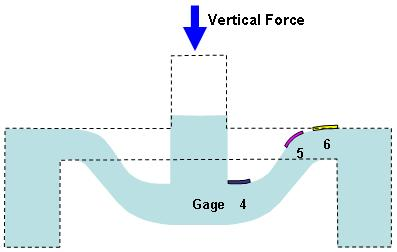Semiconductor gages are too small to cover the deforming area if you put at the end of the diaphragm. Therefore, pink gage (gage 5) detects most deformation of the diaphragm.

### Test 3: Lateral Force(leftward) Test on a Load Cell with Semiconductor Strain Gages

Instead of vertical forces, lateral forces (from the gage) are applied to the semiconductor gage load cell as shown in Figure 3-1.

• Figure 3-1: Test Setup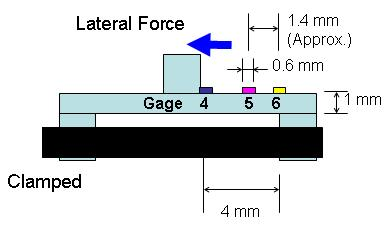• Figure 3-2: Voltage Output(mV) vs. Applied Force(N)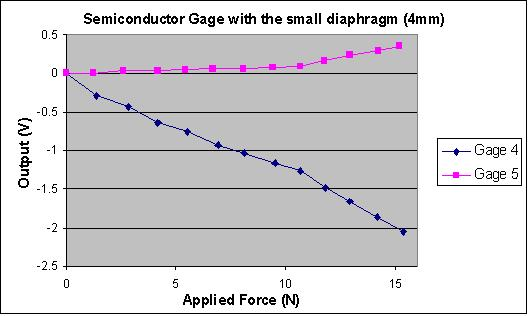Blue gage(gage 4) gives good output, but pink gage (gage 5) hardly gives output signals. This means it is very good to place a strain gage right next to the center post to detect only lateral forces. The Resulting shape is shown in Figure 3-3

• Figure 3-3: Resulting Shape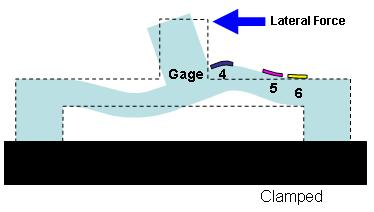### Test 4: Lateral Force(rightward) Test on a Load Cell with Semiconductor Strain Gages

The other lateral forces (to the gage) are applied to the semiconductor gage load cell as shown in Figure 4-1.

• Figure 4-1: Test Setup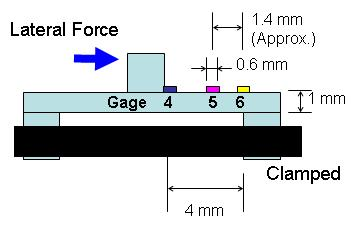• Figure 4-2: Voltage Output(mV) vs. Applied Force(N)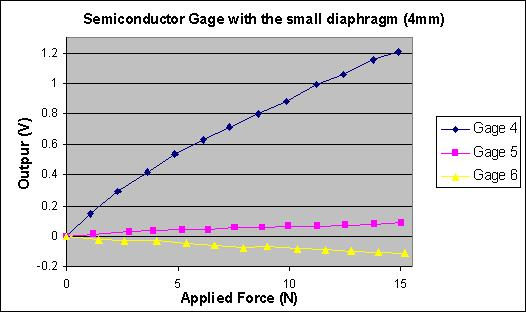The result is almost the same as that of the previous test. Only the blue gage (gagae 4) gives very good output.

• Figure 4-3: Resulting Shape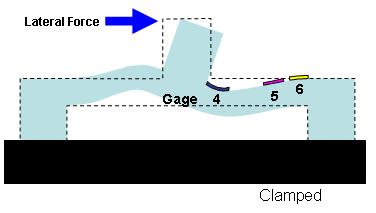Therefore, we can use the blue gage(gage 4) for lateral force detection and the pink gage (gage 5) for vertical force detection.

-- YongLaePark - 29 Aug 2005

Copyright &© by the contributing authors. All material on this collaboration platform is the property of the contributing authors.
Ideas, requests, problems regarding TWiki? Send feedback